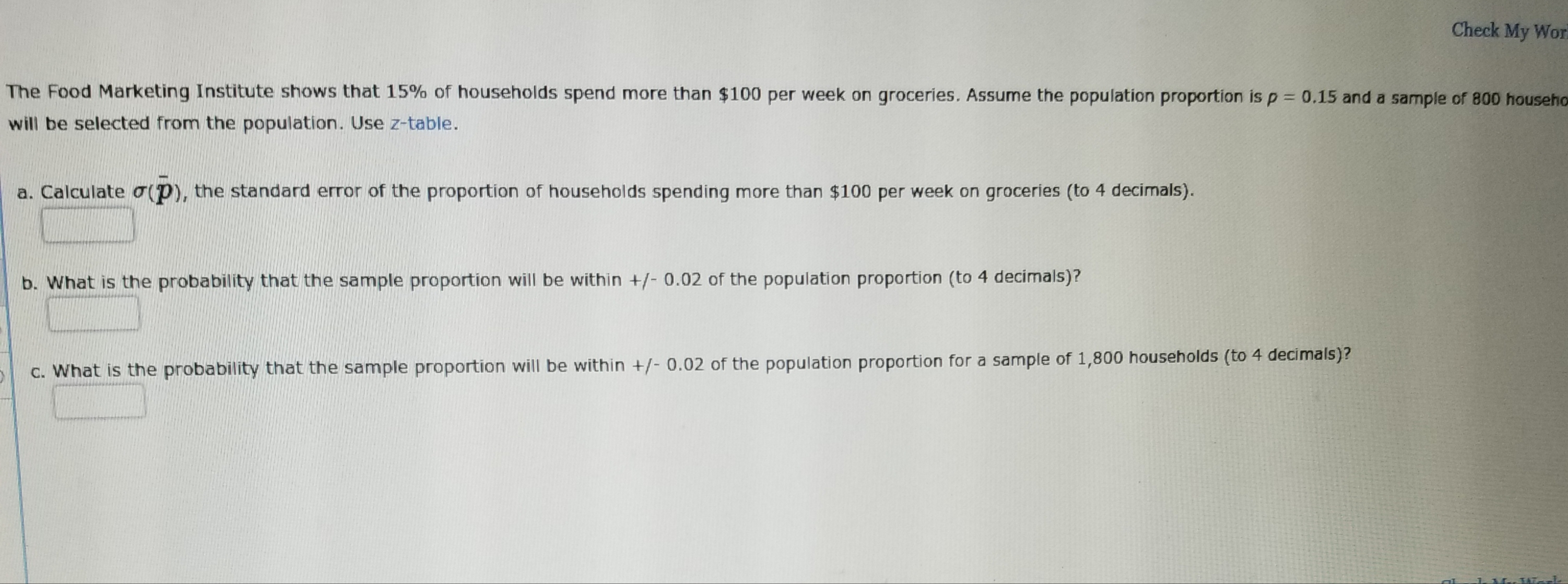# Check My WorThe Food Marketing Institute shows that 15% of households spend more than \$100 per week on groceries. Assume the population proportion is p-0.15 and a sample of 800 househowill be selected from the population. Use z-table.a. Calculate σ(P), the standard error of the proportion of households spending more than \$100 per week on groceries (to 4 decimals).b. What is the probability that the sample proportion will be within /-0.02 of the population proportion (to 4 decimals)?c. What is the probability that the sample proportion will be within +/-0.02 of the population proportion for a sample of 1,800 households (to 4 decimals)?

Question
318 viewshelp_outlineImage TranscriptioncloseCheck My Wor The Food Marketing Institute shows that 15% of households spend more than \$100 per week on groceries. Assume the population proportion is p-0.15 and a sample of 800 househo will be selected from the population. Use z-table. a. Calculate σ(P), the standard error of the proportion of households spending more than \$100 per week on groceries (to 4 decimals). b. What is the probability that the sample proportion will be within /-0.02 of the population proportion (to 4 decimals)? c. What is the probability that the sample proportion will be within +/-0.02 of the population proportion for a sample of 1,800 households (to 4 decimals)? fullscreen
check_circle

star
star
star
star
star
1 Rating
Step 1

a.

Finding the standard error of the proportion of households spending more than \$100 per week on groceries:

Here, p bar be the true population proportion of households spend more than \$100 per week on groceries, n is the sample size.

The formula for the standard error is,

Step 2

From given information p bar = 0.15 and n = 800

Then the standard error is obtained as follows:

Step 3

b.

Finding the probability that the sample proportion will be within ±0.02 of the population p...

### Want to see the full answer?

See Solution

#### Want to see this answer and more?

Solutions are written by subject experts who are available 24/7. Questions are typically answered within 1 hour.*

See Solution
*Response times may vary by subject and question.
Tagged in

### Other